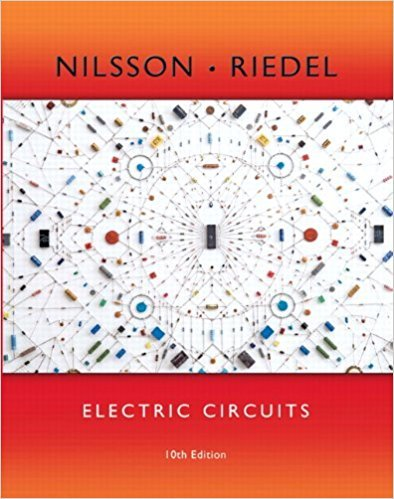×
×

# Solutions for Chapter 6.4: Mutual Inductance## Full solutions for Electric Circuits | 10th Edition

ISBN: 9780133760033Solutions for Chapter 6.4: Mutual Inductance

Solutions for Chapter 6.4
4 5 0 361 Reviews
14
4
##### ISBN: 9780133760033

This expansive textbook survival guide covers the following chapters and their solutions. This textbook survival guide was created for the textbook: Electric Circuits, edition: 10. Since 1 problems in chapter 6.4: Mutual Inductance have been answered, more than 12014 students have viewed full step-by-step solutions from this chapter. Chapter 6.4: Mutual Inductance includes 1 full step-by-step solutions. Electric Circuits was written by and is associated to the ISBN: 9780133760033.

Key Engineering and Tech Terms and definitions covered in this textbook
×